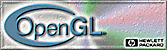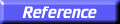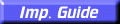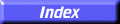# glLight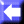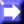glLightf, glLighti, glLightfv, glLightiv: set light source parameters.

C Specification | Parameters | Description | Notes | Errors | Associated Gets | See Also

##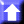C Specification

```void glLightf(
GLenum	 light,
GLenum	 pname,
GLfloat	 param)
void glLighti(
GLenum	 light,
GLenum	 pname,
GLint	 param)
void glLightfv(
GLenum	 light,
GLenum	 pname,
const GLfloat *params)
void glLightiv(
GLenum	 light,
GLenum	 pname,
const GLint *params)
```

##Parameters

light
Specifies a light. The number of lights depends on the implementation, but at least eight lights are supported. They are identified by symbolic names of the form GL_LIGHTi where 0
i < GL_MAX_LIGHTS.
pname
Specifies a single-valued light source parameter for light. GL_SPOT_EXPONENT, GL_SPOT_CUTOFF, GL_CONSTANT_ATTENUATION, GL_LINEAR_ATTENUATION, and GL_QUADRATIC_ATTENUATION are accepted.
param
Specifies the value that parameter pname of light source light will be set to.
light
Specifies a light. The number of lights depends on the implementation, but at least eight lights are supported. They are identified by symbolic names of the form GL_LIGHTi where 0i < GL_MAX_LIGHTS.
pname
Specifies a light source parameter for light. GL_AMBIENT, GL_DIFFUSE, GL_SPECULAR, GL_POSITION, GL_SPOT_CUTOFF, GL_SPOT_DIRECTION, GL_SPOT_EXPONENT, GL_CONSTANT_ATTENUATION, GL_LINEAR_ATTENUATION, and GL_QUADRATIC_ATTENUATION are accepted.
params
Specifies a pointer to the value or values that parameter pname of light source light will be set to.

##Description

glLight sets the values of individual light source parameters. light names the light and is a symbolic name of the form GL_LIGHTi, where 0i < GL_MAX_LIGHTS. pname specifies one of ten light source parameters, again by symbolic name. params is either a single value or a pointer to an array that contains the new values.

To enable and disable lighting calculation, call glEnable and glDisable with argument GL_LIGHTING. Lighting is initially disabled. When it is enabled, light sources that are enabled contribute to the lighting calculation. Light source i is enabled and disabled using glEnable and glDisable with argument GL_LIGHTi.

The ten light parameters are as follows:

GL_AMBIENT
params contains four integer or floating-point values that specify the ambient RGBA intensity of the light. Integer values are mapped linearly such that the most positive representable value maps to 1.0, and the most negative representable value maps to
1.0. Floating-point values are mapped directly. Neither integer nor floating-point values are clamped. The initial ambient light intensity is (0, 0, 0, 1).
GL_DIFFUSE
params contains four integer or floating-point values that specify the diffuse RGBA intensity of the light. Integer values are mapped linearly such that the most positive representable value maps to 1.0, and the most negative representable value maps to1.0. Floating-point values are mapped directly. Neither integer nor floating-point values are clamped. The initial value for GL_LIGHT0 is (1, 1, 1, 1); for other lights, the initial value is (0, 0, 0, 0).
GL_SPECULAR
params contains four integer or floating-point values that specify the specular RGBA intensity of the light. Integer values are mapped linearly such that the most positive representable value maps to 1.0, and the most negative representable value maps to1.0. Floating-point values are mapped directly. Neither integer nor floating-point values are clamped. The initial value for GL_LIGHT0 is (1, 1, 1, 1); for other lights, the initial value is (0, 0, 0, 0).
GL_POSITION
params contains four integer or floating-point values that specify the position of the light in homogeneous object coordinates. Both integer and floating-point values are mapped directly. Neither integer nor floating-point values are clamped.

The position is transformed by the modelview matrix when glLight is called (just as if it were a point), and it is stored in eye coordinates. If the w component of the position is 0, the light is treated as a directional source. Diffuse and specular lighting calculations take the light's direction, but not its actual position, into account, and attenuation is disabled. Otherwise, diffuse and specular lighting calculations are based on the actual location of the light in eye coordinates, and attenuation is enabled. The initial position is (0, 0, 1, 0); thus, the initial light source is directional, parallel to, and in the direction of theZ axis.

GL_SPOT_DIRECTION
params contains three integer or floating-point values that specify the direction of the light in homogeneous object coordinates. Both integer and floating-point values are mapped directly. Neither integer nor floating-point values are clamped. The spot direction is transformed by the inverse of the modelview matrix when glLight is called (just as if it were a normal), and it is stored in eye coordinates. It is significant only when GL_SPOT_CUTOFF is not 180, which it is initially. The initial direction is (0, 0,1).
GL_SPOT_EXPONENT
params is a single integer or floating-point value that specifies the intensity distribution of the light. Integer and floating-point values are mapped directly. Only values in the range [0, 128] are accepted.

Effective light intensity is attenuated by the cosine of the angle between the direction of the light and the direction from the light to the vertex being lighted, raised to the power of the spot exponent. Thus, higher spot exponents result in a more focused light source, regardless of the spot cutoff angle (see GL_SPOT_CUTOFF, next paragraph). The initial spot exponent is 0, resulting in uniform light distribution.

GL_SPOT_CUTOFF
params is a single integer or floating-point value that specifies the maximum spread angle of a light source. Integer and floating-point values are mapped directly. Only values in the range [0, 90] and the special value 180 are accepted. If the angle between the direction of the light and the direction from the light to the vertex being lighted is greater than the spot cutoff angle, the light is completely masked.

Otherwise, its intensity is controlled by the spot exponent and the attenuation factors. The initial spot cutoff is 180, resulting in uniform light distribution.

GL_CONSTANT_ATTENUATION, GL_LINEAR_ATTENUATION , GL_QUADRATIC_ATTENUATION
params is a single integer or floating-point value that specifies one of the three light attenuation factors. Integer and floating-point values are mapped directly. Only nonnegative values are accepted. If the light is positional, rather than directional, its intensity is attenuated by the reciprocal of the sum of the constant factor, the linear factor times the distance between the light and the vertex being lighted, and the quadratic factor times the square of the same distance. The initial attenuation factors are (1, 0, 0), resulting in no attenuation.

##Notes

It is always the case that GL_LIGHTi = GL_LIGHT0 + i.

##Errors

• GL_INVALID_ENUM is generated if either light or pname is not an accepted value.
• GL_INVALID_VALUE is generated if a spot exponent value is specified outside the range [0,128], or if spot cutoff is specified outside the range [0,90] (except for the special value 180), or if a negative attenuation factor is specified.
• GL_INVALID_OPERATION is generated if glLight is executed between the execution of glBegin and the corresponding execution of glEnd.

##Associated Gets

glGetLight
glIsEnabled with argument GL_LIGHTING

glColorMaterial,
glLightModel,
glMaterial